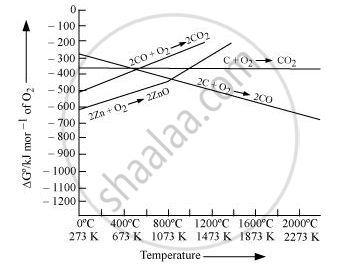Share

# Out of C and CO, which is a better reducing agent for ZnO ? - CBSE (Science) Class 12 - Chemistry

ConceptExtraction of Crude Metal from Concentrated Ore

#### Question

Out of C and CO, which is a better reducing agent for ZnO ?

#### Solution 1Reduction of ZnO to Zn is usually carried out at 1673 K. From the above figure, it can be observed that above 1073 K, the Gibbs free energy of formation of CO from C and above 1273 K, the Gibbs free energy of formation of CO2 from C is lesser than the Gibbs free energy of formation of ZnO. Therefore, C can easily reduce ZnO to Zn.

On the other hand, the Gibbs free energy of formation of CO2 from CO is always higher than the Gibbs free energy of formation of ZnO. Therefore, CO cannot reduce ZnO. Hence, C is a better reducing agent than CO for reducing ZnO.

#### Solution 2

The two reduction reactions are :

ZnO(s) + C(s) -> Zn(s) + CO(g) ... (i)

ZnO(s) + CO(g) -> Zn(s) + CO_2(g) .... (ii)

In the first case, there is increase in the magnitude of ΔS° while in the second case, it almost remains the same. In other words ΔG° will have more negative value in the first case when C(s) is used as the reducing agent than in the second case when CO(g) acts as the reducing agent. Therefore, C(s) is a better reducing agent.

Is there an error in this question or solution?

#### APPEARS IN

NCERT Solution for Chemistry Textbook for Class 12 (2018 to Current)
Chapter 6: General Principles and Processes of Isolation of Elements
Q: 22 | Page no. 164

#### Video TutorialsVIEW ALL 

Solution Out of C and CO, which is a better reducing agent for ZnO ? Concept: Extraction of Crude Metal from Concentrated Ore.
S